NCERT Solutions for Class 9 Maths Chapter 11 Circles Ex 11.6 are part of NCERT Solutions for Class 9 Maths. Here we have given NCERT Solutions for Class 9 Maths Chapter 11 Circles Ex 11.6.

 Board CBSE Textbook NCERT Class Class 9 Subject Maths Chapter Chapter 11 Chapter Name Circles Exercise Ex 11.6 Number of Questions Solved 10 Category NCERT Solutions

## NCERT Solutions for Class 9 Maths Chapter 11 Circles Ex 11.6

Question 1.
Prove that the line of centres of two intersecting circles subtends equal angles at the two points of intersection.
Solution:
Given: Two circles with centres O and O’ which intersect each other at C and D.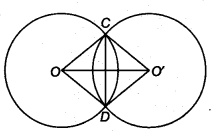To prove: ∠OCO’ = ∠ODO’
Construction: Join OC, OD, O’C and O’D
Proof: In ∆ OCO’and ∆ODO’, we have
OC = OD (Radii of the same circle)
O’C = O’D (Radii of the same circle)
OO’ = OO’ (Common)
∴ By SSS criterion, we get
∆ OCO’ ≅ ∆ ODO’
Hence, ∠OCO’ = ∠ODO’ (By CPCT)

Question 2.
Two chords AB and CD of lengths 5 cm and 11 cm, respectively of a circle are parallel to each other and are on opposite sides of its centre. If the distance between AB and CD is 6 cm, find the radius of the circle.
Solution:
Let O be the centre of the given circle and let its radius be cm.
Draw ON ⊥ AB and OM⊥ CD since, ON ⊥ AB, OM ⊥ CD and AB || CD, therefore points N, O, M are collinear.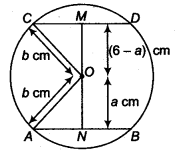Let ON = a cm
∴ OM = (6 – a) cm
Join OA and OC.
Then, OA = OC = b c m
Since, the perpendicular from the centre to a chord of the circle bisects the chord.
Therefore, AN = NB= 2.5 cm and OM = MD = 5.5 cm
In ∆OAN and ∆OCM, we get
OA2 = ON2 + AN2
OC2 = OM2 + CM2
⇒ b2 = a2 + (2.5)2
and, b2 = (6-a)2 + (5.5)2 …(i)
So, a2 + (2.5)2 = (6 – a)2 + (5.5)2
⇒ a2 + 6.25= 36-12a + a2 + 30.25
⇒ 12a = 60
⇒ a = 5
On putting a = 5 in Eq. (i), we get
b2 = (5)2 + (2.5)2
= 25 + 6.25 = 31.25
So, r = $$\sqrt{31.25}$$ = 5.6cm (Approx.)

Question 3.
The lengths of two parallel chords of a circle are 6 cm and 8 cm. If the smaller chord is at distance 4 cm from the centre, what is the distance of the other chord from the centre ?
Solution:
Let PQ and RS be two parallel chords of a circle with centre O such that PQ = 6 cm and RS = 8 cm.
Let a be the radius of circle.Draw ON ⊥ RS, OM ⊥ PQ. Since, PQ || RS and ON ⊥ RS, OM⊥ PQ, therefore points 0,N,M are collinear.
∵ OM = 4 cm and M and N are the mid-points of PQ and RS respectively.
PM = MQ = $$\frac { 1 }{ 2 }$$ PQ = $$\frac { 6 }{ 2 }$$ = 3 cm
and RN = NS = $$\frac { 1 }{ 2 }$$ RS = $$\frac { 8 }{ 2 }$$ = 4 cm
In ∆OPM, we have
OP2 = OM2 + PM2
⇒ a2 =42 + 32 = 16 + 9 = 25
⇒ a = 5
In ∆ORN, we have
⇒ OR2 = ON2 + RN2
⇒ a2 = ON2 + (4)2
⇒ 25 = ON2 + 16
⇒ ON2 = 9
⇒ ON = 3cm
Hence, the distance of the chord PS from the centre is 3 cm.

Question 4.
Let the vertex of an angle ABC be located outside a circle and let the sides of the angle intersect equal chords AD and CE with the circle. Prove that ∠ABC is equal to half the difference of the angles subtended by the chords AC and DE at the centre.
Solution:
Since, an exterior angle of a triangle is equal to the sum of the interior opposite angles.∴ In ∆BDC, we get
∠ADC = ∠DBC + ∠DCB …(i)
Since, angle at the centre is twice at a point on the remaining part of circle.
∴ ∠DCE = $$\frac { 1 }{ 2 }$$ ∠DOE
⇒ ∠DCB = $$\frac { 1 }{ 2 }$$ ∠DOE (∵ ∠DCE = ∠DCB)
∠ADC = $$\frac { 1 }{ 2 }$$ ∠AOC
∴ $$\frac { 1 }{ 2 }$$ ∠AOC = ∠ABC + $$\frac { 1 }{ 2 }$$ ∠DOE (∵ ∠DBC = ∠ABC)
∴ ∠ABC = $$\frac { 1 }{ 2 }$$ (∠AOC – ∠DOE)
Hence, ∠ABC is equal to half the difference of angles subtended by the chords AC and DE at the centre.

Question 5.
Prove that the circle drawn with any side of a rhombus as diameter, passes through the point of intersection of its diagonals.
Solution:
Given: PQRS is a rhombus. PR and SQ are its two diagonals which bisect each other at right angles.
To prove: A circle drawn on PQ as diameter will pass through O.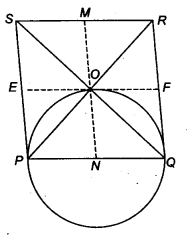Construction: Through O, draw MN || PS and EF || PQ.
Proof : ∵ PQ = SR ⇒ $$\frac { 1 }{ 2 }$$ PQ = $$\frac { 1 }{ 2 }$$ SR
So, PN = SM
Similarly, PE = ON
So, PN = ON = NQ
Therefore, a circle drawn with N as centre and radius PN passes through P, O, Q.

Question 6.
ABCD is a parallelogram. The circle through A, B and C intersect CD (produced if necessary) at E. Prove that AE = AD.
Solution:
Since, ABCE is a cyclic quadrilateral, therefore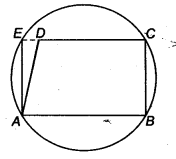∠AED+ ∠ABC= 180°
(∵ Sum of opposite angle of a cyclic quadrilateral is 180°) .. .(i)
So, ∠ADE + ∠ABC = 180°
(∵ ∠ADC = ∠ABC opposite angle of a || gm).. .(ii)
From Eqs. (i) and (ii), we get
∠AED + ∠ABC = ∠ADE + ∠ABC
∴ In ∆AED We have
(∵ Sides opposite to equal angles of a triangle are equal)

Question 7.
AC and BD are chords of a circle which bisect each other. Prove that
(i) AC and BD are diameters,
(ii) ABCD is a rectangle.
Solution:
(i) Let BD and AC be two chords of a circle bisect at P.In ∆APB and ∆CPD, we get
PA = PC ( ∵ P is the mid-point of AC)
∠APB = ∠CPD (Vertically opposite angles)
and PB = PD (∵ P is the mid-point of BD)
∴ By SAS criterion
∆CPD ≅ ∆APB
∴ CD= AB (By CPCT) …(i)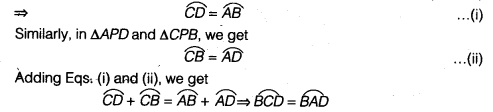∴ BD divides the circle into two equal parts. So, BD is a diameter.
Similarly, AC is a diameter.
(ii) Now, BD and AC bisect each other.
So, ABCD is a parallelogram.
Also, AC = BD
∴ ABCD is a rectangle.

Question 8.
Bisectors of angles A, B and C of a ∆ABC intersect its circumcircle at D, E and F, respectively. Prove that the angles of the ∆DEF are 90° – $$\frac { 1 }{ 2 }$$ A, 90° – $$\frac { 1 }{ 2 }$$ B and 90° – $$\frac { 1 }{ 2 }$$ C.
Solution:
∵ ∠EDF = ∠EDA + ∠ADF
∵ ∠EDA and ∠EBA are the angles in the same segment of the circle.
∴ ∠EDA = ∠EBA
and similarly ∠ADF and ∠FCA are the angles in the same segment and hence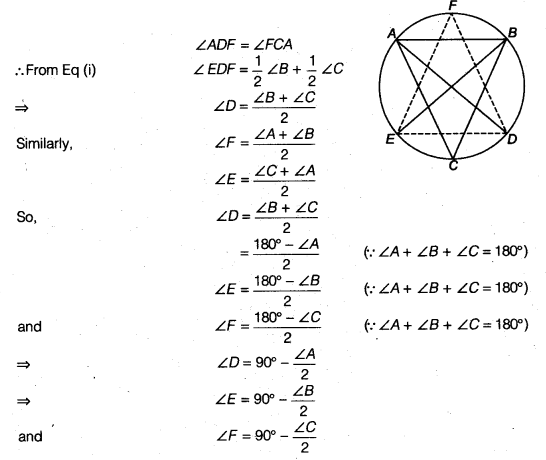Question 9.
Two congruent circles intersect each other at points A and B. Through A any line segment PAQ is drawn so that P, Q lie on the two circles. Prove that BP = BQ.
Solution:
Let O’ and O be the centres of two congruent circles.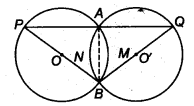Since, AB is a common chord of these circles.
∴ ∠BPA = ∠BQA
(∵ Angle subtended by equal chords are equal)
⇒ BP = BQ

Question 10.
In any ∆ ABC, if the angle bisector of ∠A and perpendicular bisector of BC intersect, prove that they intersect on the circumcircle of the ∆ABC.
Solution:
(i) Let bisector of ∠A meet the circumcircle of ∆ABC at M.
Join BM and CM.∴ ∠MBC = ∠MAC (Angles in same segment)
and ∠BCM = ∠BAM (Angles in same segment)
But ∠BAM = ∠CAM (∵ AM is bisector of ∠A)…. .(i)
∴ ∠MBC = ∠BCM
So, MB = MC (Sides opposite to equal angles are equal)
So, M must lie on the perpendicular bisector of BC
(ii) Let M be a point on the perpendicular bisector of BC which lies on circumcircle of ∆ ABC.
Join AM.Since, M lies on perpendicular bisector of BC.
∴ BM = CM
∠MBC = ∠MCB
But ∠MBC = ∠MAC (Angles in same segment)
and ∠MCB = ∠BAM (Angles in same segment)
So, from Eq. (i),
∠BAM = ∠CAM
AM is the bisector of A.
Hence, bisector of ∠A and perpendicular bisector of BC at M which lies on circumcircle of ∆ABC.
We hope the NCERT Solutions for Class 9 Maths Chapter 11 Circles Ex 11.6, help you. If you have any query regarding NCERT Solutions for Class 9 Maths Chapter 11 Circles Ex 11.6, drop a comment below and we will get back to you at the earliest.